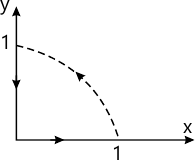Total marks: --
Total time: --
INSTRUCTIONS
(1) Assume appropriate data and state your reasons
(2) Marks are given to the right of every question
(3) Draw neat diagrams wherever necessary

1(a) State vector form of Coulomb's law of force between two point charges and indicate the units of the quantities in the equation.
6 M
1(b) State and prove Gauss law for point charge.
6 M
1(c) Two point charges,Q1 and Q2 are located at (1,2,0)m and (2,0,0)m respectively.Find the relation between the charges Q1 and Q2 such that the total force on a unit positive charge at (-1,1,0) have i) no x-component.
8 M

2(a) Define potential difference and absolute potential.
4 M
2(b) Establish the relation $E=\bigtriangleup \vee.$
6 M
2(c) Electrical potential at an arbitrary point free - space is given as :
$V-(x+1)^{2}+(y+2)^{2}+(z-3)^{2}. At \ P (2,1,0)\ find\\ i) v\ ii) E^{\rightarrow }\ iii) E^{\rightarrow }\ iv) D^{\rightarrow }\ v) D^{\rightarrow }\ vi) P_{v}.$
10 M

3(a) Derive the expression for Poisson's equation.
4 M
3(b) Write the expression for Laplace's equation in cylindrical and spherical coordinates.
4 M
3(c) State and prove uniqueness theorem.
6 M
3(d) Given the potential field$V=x^{2}yz-ky^{3}z$ volts :
i) Find k if potential field satisfies Laplace's equation
ii) find$E^{\rightarrow }$at (1,2,3).
6 M

4(a) Starting form Biot-Savort's law, derive the expression for the magnetic field intensity at a point due to finite length current carrying conductor.
8 M
4(b) Verify stoke's theorem for the field $\underset{H}{\rightarrow}$_$2rcos\Theta a\ r^{\vee }+ra\Theta ^{\wedge }$ for the path shown r=0 to 1; 0 to $90^{0}$8 M
4(c) Explain scalar and vector magnetic potenial.
4 M

5(a) Derive expression for magnetic force on :
i) Moving point charge
Differential current element.
10 M
5(b) A current element$I_{1}dI_{2}-10^{-4}\ \widehat{a_z}$ (AM) is located at$P_{1}$(-2,0,0).Both are in free space :
Find force exerted on $I_{2}d1_{2}-10^{-6}[{\widehat{ax}}-2\widehat{ay}+3\widehat{az}](Am)$ is located at $P_{2}$(-2,0,0). Both are in free space:
i) Find force exerted on $I_{2}dl_{2}by I_{2}dI_{1}$
ii) Find force exerted on$I_{1}dl_{1}by I_{2}dI_{2}.$
10 M

6(a) List Maxwell's equations in point form and lntergral form.
8 M
6(b) A homogeneous material has ?=2×1$\epsilon =2×10^{6} F/M and\ \mu =1.25×10^{5}$ and$\sigma =0.$.Electric field intensity $\overrightarrow{E}$=400 cos$(10^{9}t-kz)a\widehat{x}\ V/m$. If all the field vary sinsoidally,find$\overrightarrow{D},\overrightarrow{B},\overrightarrow{H} and k using Maxwell's equations. 12 M 7(b) State and explain Poynting theorem. 10 M 7(c) Starting form Maxwell's equations derive wave equation in E and H for a uniform plane wave travelling in free space. 10 M 8(a) Write short notes on : i) SWR and reflection coefficient ii) Skin depth. 10 M 8(b) A Ghz plane wave in free space has electric field intensity 15 V/m. Find: i) Velocity of propagation ii) Wavelength iii) Characteristic impedance of the medium iv) Amplitude of magnetic field intensity v) Propagation constant\[\beta$.
10 M

More question papers from Field Theory